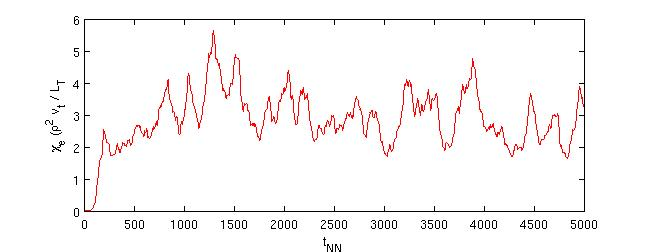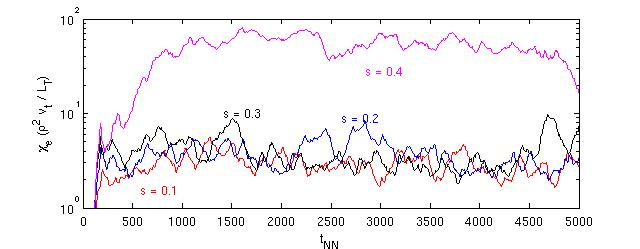# Gyro-Kinetics @ Warwick

GKW stands for Gyro-Kinetics@Warwick. It is both the name of a projects as well as a computer code. GKW solves the nonlinear gyro-kinetic equations in a 3D flux tube geometry including general geometry, an arbitrary number of species, electro-magnetic effects, and collisions.

The aim of the research project is to accurately assess the magnitude of the turbulent fluxes of particles , energy and momentum in a tokamak. The larger framework is, therefore, the research on nuclear fusion, a possible alternative energy source. There is more than one approach to fusion, but the most developed is the aproach based on magnetic confinent, with the tokamak being the most developed device.

• If the above is spanish to you, then go here for a brief description
• Online documentation can be found here
• For examples / benchmarks go here
• Publications as well as research results can be found here
• If all of this convinces you and you want to run the code go to the download page

As an example of the solutions obtained, we show here the ETG benchmark of Nevins. Shown is the electron heat conduction coefficient as a function of normalized time (t v_th / L_t) for cyclone base case parameters (q = 1.4, eps = 0.19, R/L_t = 6.9, R/Ln = 2.2) with the value of the magnetic shear changed compared with the Cyclone base case to shat = 0.1. The Nevins paper gives a value 2.95 +/- 0.15 for this case, based on GYRO and GENE simulations. GKW yields a value 3.0 +/- 0.2The shear value was changed to 0.1 in the Nevins benchmark case since it was found that for shat > 0.3 there is no converged case. GKW finds the same dependence on the magnetic shear as shown in the next graph